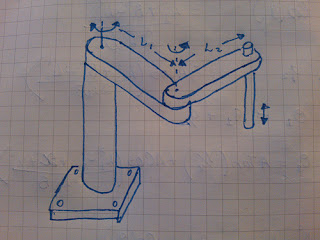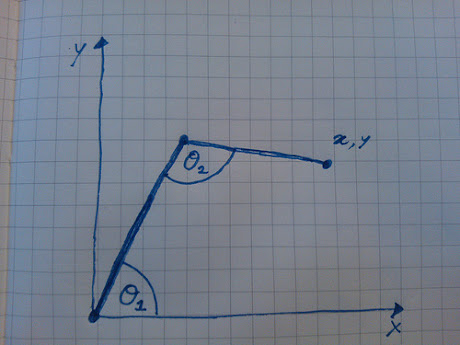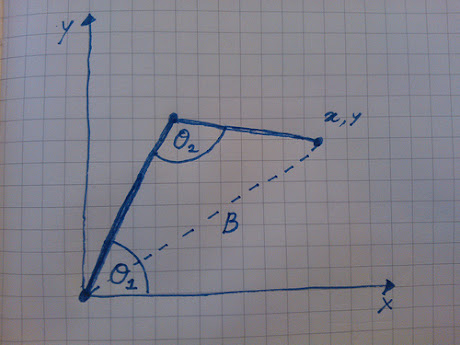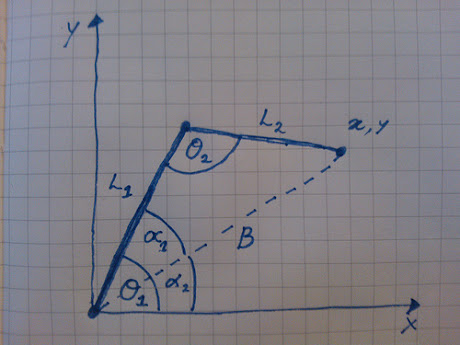# Inverse Kinematics and robot arms

Posted September 25 2012, tagged math, robots,

I have recently been thinking about robotic arms.  Specifically how to move the an articulated arm so that the end is at a particluar location.  I was aware that this is covered within the topic of Inverse Kinematics, but I had'nt really looked in to it before.Following the very good explanations on Learn about Robotics I realised that it is'nt too hard to.  However the author skips a few points in deriving the formula, and I wanted to get a complete understanding on how its done.

This is for a particular case of robots where the movement of the arms is in two-dimensions, know as a SCARA robot.  The image on the right shows a sketch of this. Each arm can rotate around one axis (i.e. they can't move up and down).  It is usually the end effector that can move up and down.

The problem comes down to calculating what angle to rotate each arm so that the end point is above a fixed point in space.

If we simplify the diagram and draw the arms from the top view we describe the problem using something like the following graph.  The x1,y1 is the target location, we know the length of each arm, it is the angles θ1 and θ2 which need to be calculatedThe first stage is to plot a line from the origin to the desired x,y point to form a triangle. We will call this line B.Adding in the various component angles and known quantities, we have a problem to solve that appears as follows;## Equations to know and understand

The following equations are used to solve this problem;

Pythagoras theorem - the square of the hyponenus is equal to the sum of the squares of the other two sides. a2 = b2 + c2

Law of Cosines - Relates the lengths of the sides (of a triangle) to the cosine of one of its angles. a2 = b2 + c2 -2bc * Cos(α)

Trigonometry - Relationships between an angle and the length of two sides of a triangle i.e. SOH, TOA, CAH

## Calculating Inverse Kinematics

Given what we now know, the calculation of α1 and &alpha2; is a case of solving the equations. So here are all the steps needed to get to the two equations.

B2 = L12 + L22
∴ B = √(x2 + y2)

Tan(q1) = y / x
∴ q1 = ATan(y/x)

Taking the Law of Cosines;
L22 = L12 + B2 - 2 * L1 * B * Cos(q2)
L12 + B2 + L22 = 2 * L1 * B * Cos(q2)
Cos(q2) = ( (L12 + B2 + L22) / (L1 * B) )
∴ q2 = ACos( (L12 + B2 + L22) / (L1 * B) )

Since θ1 = q1 + q2
∴ θ1 = ATan(y / x) + ACos( (L12 + B2 + L22) / (L1 * B) )

Using the Law of Cosines again
B2 = L12 + L22 - 2 * L1 * L2 * Cos(θ2)
B2 - L12 - L22 = 2 * L1 * L2 * Cos(θ2)
Cos(θ2) = ( (B2 - L12 - L22) / (2 * L1 * L2) )
∴ θ2 = ACos ( (B2 - L12 - L22) / (2 * L1 * L2) )

## Summary

To calculate the angles needed for a SCARA robot to have its end effector at a certain location use the following equations;
θ1 = ATan(y / x) + ACos( (L12 + B2 + L22) / (L1 * B) )
θ2 = ACos ( (B2 - L12 - L22) / (2 * L1 * L2) )

I hope that all makes sense?

## Edit

I've written a demo canvas toy that demonstrates inverse kinematics in action.  This uses the equations shown above to move the arm to a desired location.